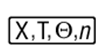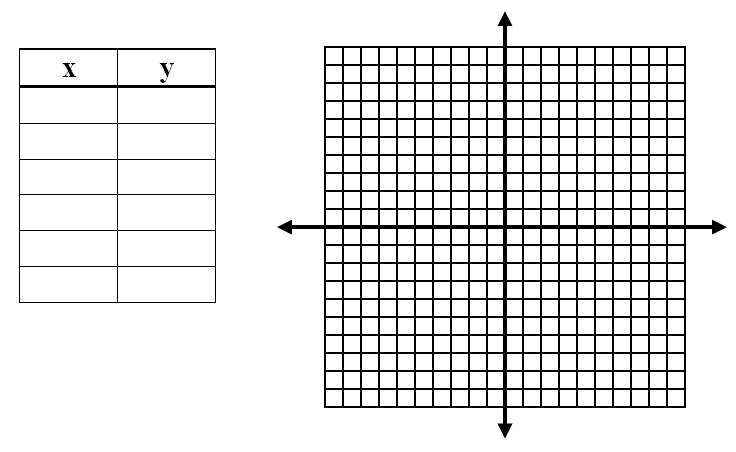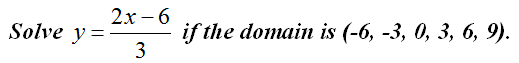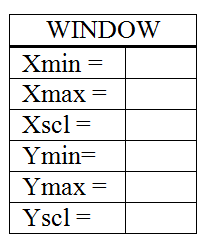# Use a TI Graphing Calculator ‘s Table

//Use a TI Graphing Calculator ‘s Table
Use a TI Graphing Calculator ‘s Table 2017-11-13T22:01:52+00:00

You can use your graphing calculator to not only graph equations of lines, but also to generate a table of data.

1. Make sure that your calculator has the Plots Off, Y= functions cleared, the MODE and FORMAT are set at “stage left”, and the lists are cleared.
2. Press the Y= button. With your cursor in the Y1 line, type 2+3 then Enter You should have the equation y = 2x + 3 entered into the calculator.
3. Press the ZOOM key.  Choose option 6 for ZStandard.  This gives you a coordinate plane with –10 to 10 as its x and y values.
4. Press the 2nd button, then TABLE (shift of GRAPH) to see a table of values for your equation.
5. Fill out the table below with 6 values for x and y.
6. Press the GRAPH button to see the line again and sketch what you see on the calculator on the coordinate plane.1. Repeat the process with the equation 4x + y = -5.2. Type the equation y=(2x – 6)/3 in Y1.  Press 2nd then TBLSET (shift of WINDOW). Change TblStart = to -6 and ΔTBl = to 3.  Press 2nd then TABLE to view the values for x and y.  Record your table below.3. Why did 2x-6 have to be in parenthesis in number 8?
4. Why did we choose ΔTBl = to be equal to 3?

A printable version of this activity is available for classroom use. Tables on TI Graphing Calculators